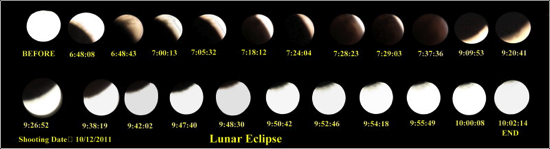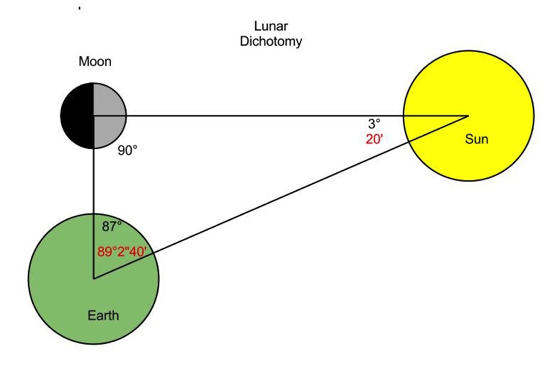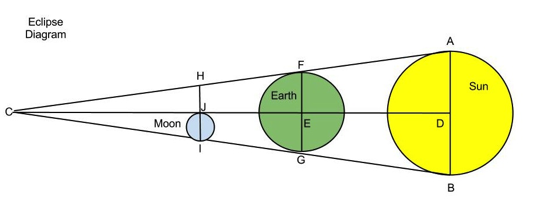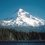# Lunar EclipsesLunar Eclipse

Last week we wrote about how the Ancient Greeks cleverly made a measurement of the absolute size of the Earth. Impressively, they also attempted an estimate of the scale of the Sun and Moon. Aristarchus of Samos (310-230 BC), used lunar eclipses to estimate the sizes of, and distances between, the Earth, Moon, and Sun. Aristarchus is also famous for being the first person to propose a heliocentric theory of the solar system. Unfortunately, his writings on heliocentric theory have been lost to history. We know of their existence only through the commentary of his critics. To estimate the scale of the Sun and the Moon, Aristarchus needed simple geometry, simple spatial reasoning, and observations that are impossible to make. His geometry was correct and clever, but his conclusions were hopelessly inaccurate.

In his treatise, On the Sizes and Distances (of the Sun and Moon), Aristarchus first set out to find the relative distance of the Moon to the Earth and the Earth to the Sun. He did this by conceiving of the lunar dichotomy diagram (below). He reasoned that at the precise moment when the Moon is a quarter Moon, and the dark side of the Moon is visible as a perfect half circle, that a right triangle can be constructed between the Moon, Earth, and Sun. With a measure of the angular separation in the sky between a daytime quarter Moon and the Sun, Aristarchus could use the angle to find the ratios of the sides of the triangle to each other.Lunar Dichotomy

Measuring this angle between the Moon and the Sun with the naked eye, with any meaningful precision is a hopeless task. This would be the weakest link of Aristachus’s estimate of the scale of the solar system. No matter how correct his subsequent geometry is, being off by a few degrees translates into huge errors across the large scale of the solar system. The actual angle is about 89° 2” 20’, which is too close to a right angle for humans to realistically perceive. Aristarchus did not let his inability to measure this angle prevent him for from trying out a number. He reckoned, chose, or inaccurately measured this angle to be 87°.

Today we have calculators with trig functions. Our grandfathers had large books of tables containing the sine, cosine, and tangent ratios of various angles. Aristarchus had non of these. Today we would simply use our calculators to find the cosecant of 3°, and find that Aristarchus thought the Sun to be 19.1 times as far away from the Earth as the Moon. Aristarchus did not have a calculator. Through a laborious procedure, in a era when scratch paper and pens were not as handy as they are today, he determined that the Sun was greater than 18 times as far away from the Earth as the Moon was, but less than 20. Modern measurements place the Sun's distance from Earth at about 390 times the Moons distance from the Earth. Oops... oh well.

Through a happy coincidence, Aristarchus was then able to estimate the relative sizes of the Sun and the Moon. A basic fact about most visual perception, is that the size we perceive an object to be, is inversely proportional to it’s distance from us. The size that objects appear to us is referred to as their angular size, or visual angle. If you know an object’s actual size, you can use its angular size to derive its distance. Similarly, if you know an objects distance, you can use its angular size to calculate its actual size. From observing full solar eclipses, when the Moon perfectly covers the Sun, the Greeks decided that the Sun and the Moon conveniently had the exact same apparent diameter (this is close to true). Aristarchus reasoned that the only way the Sun and the Moon could have the same apparent size, was if their difference in size was exactly proportional to their differences in distance from the Earth. He therefore concluded that the Sun was 18-20 times larger than the moon.

Aristarchus now thought he had the relative sizes and distances of the Sun and the Moon, but this still didn’t solve an ambiguity of whether they were huge and far away, or smaller and close to earth. To resolve this, he had to find a way to express these relative sizes and distances in terms of the Earth’s radius. Aristarchus discovered a really slick way of doing this using the geometry of lunar eclipses.

During a full lunar eclipse, the Moon passes into the Earth’s shadow, and is occluded from the Sun's illumination. In an eclipse, the Sun, Earth, and Moon fall along a line. This orientation was apparent to the Ancient Greeks, whether they believed in a geocentric solar system or a heliocentric one. The dark part of the Earth’s shadow is known as the umbra, and forms a cone extending from the dark side of the Earth. The sides of this shadow cone comprise lines projecting tangent to the spheres of the Earth and the Sun.

While observing a lunar eclipse, Aristarchus measured the diameter of the Earth’s shadow cone to be twice the diameter of the Moon, at the distance of the Moon. He likely measured this using a water clock to time the passage of the moon, into and out of, the Earth's shadow cone. He could have measured the time elapsed from the moment when the Moon's edge first entered the umbra, to the moment when the moon completely entered the shadow. He then could have measured the time it took for the first sliver of the moon to become visible at the other side of the umbra. If he assumed that the moon orbits at a constant angular velocity, and then divided the time that the moon spent entirely in the shadow by the time it took the moon to enter the shadow, he could have found the width of the shadow in moon diameters. He used this to construct the eclipse diagram below, where triangle FCG is the Earth's umbra.Diagram of the Eclipse

$\frac{AB}{JI} = \frac{18}{1}$

$HI = 2JI$

That would make $HI$ $\frac{1}{9}$ of $AB$. By the similarity of triangles ACB and HCI, that would make the distance of $DC$ 9 times greater than $JC$.

$\frac{HI}{AB} = \frac{1}{9}$

$\frac{DC}{JC} = \frac{9}{1}$

From this, $\frac{DC}{DJ}$ would be $\frac{9}{8}$. If the Sun is 18 times further away from the Earth than the Moon is, when the Moon is on the opposite side of the Earth from the Sun, it is an additional $\frac{1}{18}$ the Earth-Sun distance from the Sun. So:

$\frac{DC}{DJ} = \frac{9}{8}$

$\frac{DJ}{DE} = \frac {19}{18}$

What he wanted was the ratio of $\frac{DC}{DE}$ so that by comparing similar triangles he could get $\frac{AB}{FG}$. From the relationships above:

$DC = DJ \frac{9}{8}$

$DE = DJ \frac{18}{19}$

$\frac{DC}{DE} = \frac{9\times19}{8\times 18} = \frac{19}{16}$

From the similarity of triangles ACB and FCG he found the Earth to be at most $\frac{3}{19}$ the size of the sun and about 3 times larger than the moon(60/19).

To express these sizes and distances in terms of Earth radii, all he now needed was a measure of the apparent size of the Sun and the Moon. Here, Aristarchus gets confusing. Later sources suggest that Aristarchus measured the apparent diameter of the Sun and Moon to be $\frac{1}{720}$ of their orbit, or $\frac{1}{2}$ degree. This would be close to accurate. However, in his treatise On the Sizes and Distances of the Moon and the Sun, he choose 2 degrees, which is way too large.

If the Moon is 1/3 the diameter of the earth, then the Moon’s diameter is 2/3 of the Earth’s radius. Using an apparent diameter of 2 degrees, the circumference of the Moon's orbit would then be $180 \times \frac{2}{3} =$ 120 e.r. The distance to the Moon, would then be the radius of this orbit, or $\frac{120}{2\pi}$ which is about 19 e.r. The distance to the Sun would then be 18 times this, or about 343 e.r. Our modern measurements establish the Earth-Sun distance as 23,500 e.r., and the Moon-Earth distance at about 60 e.r. So he was a little off...modern measurements put the Suns diameter at 110 times the Earth's, and the Earth's diameter at about 3.8 times the moons.

Later Greek astronomer's would use the eclipse diagram in both the same way, and slightly different ways, to refine estimates for the scale of the solar system. Using a much more reasonable angular diameter for the Sun and Moon of $\frac{1}{2}$ degree, you get more reasonable estimates for the lunar distance, but are still hopelessly off of the solar one. Aristarchus's successors were able to use the eclipse diagram to extend the solar distance to about 1,200 e.r. Humankind's understanding of the scale of the solar system would be dependent on the eclipse diagram, and remain in this order of magnitude for another 2,000 years. It was not until telescopes in the 17th century made new phenomena visible and measurable, that new and better ways to estimate the size of the solar system were discovered.

Information on Aristarchus's method of calculation was sourced from:

Measuring the Universe: Cosmic Dimensions from Aristarchus to Halley, by Albert Van Helden. University of Chicago Press, 1985.

The ancient greek sections are from p.1-40 if you would like to get a peak at some of the book on Google books preview.Note by Peter Taylor
7 years, 3 months ago

This discussion board is a place to discuss our Daily Challenges and the math and science related to those challenges. Explanations are more than just a solution — they should explain the steps and thinking strategies that you used to obtain the solution. Comments should further the discussion of math and science.

When posting on Brilliant:

• Use the emojis to react to an explanation, whether you're congratulating a job well done , or just really confused .
• Ask specific questions about the challenge or the steps in somebody's explanation. Well-posed questions can add a lot to the discussion, but posting "I don't understand!" doesn't help anyone.
• Try to contribute something new to the discussion, whether it is an extension, generalization or other idea related to the challenge.
• Stay on topic — we're all here to learn more about math and science, not to hear about your favorite get-rich-quick scheme or current world events.

MarkdownAppears as
*italics* or _italics_ italics
**bold** or __bold__ bold
- bulleted- list
• bulleted
• list
1. numbered2. list
1. numbered
2. list
Note: you must add a full line of space before and after lists for them to show up correctly
paragraph 1paragraph 2

paragraph 1

paragraph 2

[example link](https://brilliant.org)example link
> This is a quote
This is a quote
    # I indented these lines
# 4 spaces, and now they show
# up as a code block.

print "hello world"
# I indented these lines
# 4 spaces, and now they show
# up as a code block.

print "hello world"
MathAppears as
Remember to wrap math in $$ ... $$ or $ ... $ to ensure proper formatting.
2 \times 3 $2 \times 3$
2^{34} $2^{34}$
a_{i-1} $a_{i-1}$
\frac{2}{3} $\frac{2}{3}$
\sqrt{2} $\sqrt{2}$
\sum_{i=1}^3 $\sum_{i=1}^3$
\sin \theta $\sin \theta$
\boxed{123} $\boxed{123}$

## Comments

Sort by:

Top Newest

Too lazy!

- 6 years, 6 months ago

Log in to reply

×

Problem Loading...

Note Loading...

Set Loading...